Thursday, July 22, 2010

Problem Reduction with AO* Algorithm.

PROBLEM REDUCTION ( AND - OR graphs - AO * Algorithm)

When a problem can be divided into a set of sub problems, where each sub problem can be solved separately and a combination of these will be a solution, AND-OR graphs or AND - OR trees are used for representing the solution. The decomposition of the problem or problem reduction generates AND arcs. One AND are may point to any number of successor nodes. All these must be solved so that the arc will rise to many arcs, indicating several possible solutions. Hence the graph is known as AND - OR instead of AND. Figure shows an AND - OR graph.

An algorithm to find a solution in an AND - OR graph must handle AND area appropriately. A* algorithm can not search AND - OR graphs efficiently. This can be understand from the give figure.
FIGURE : AND - OR graph

In figure (a) the top node A has been expanded producing two area one leading to B and leading to C-D . the numbers at each node represent the value of f ' at that node (cost of getting to the goal state from current state). For simplicity, it is assumed that every operation(i.e. applying a rule) has unit cost, i.e., each are with single successor will have a cost of 1 and each of its components. With the available information till now , it appears that C is the most promising node to expand since its f ' = 3 , the lowest but going through B would be better since to use C we must also use D' and the cost would be 9(3+4+1+1). Through B it would be 6(5+1).

Thus the choice of the next node to expand depends not only n a value but also on whether that node is part of the current best path form the initial mode. Figure (b) makes this clearer. In figure the node G appears to be the most promising node, with the least f ' value. But G is not on the current beat path, since to use G we must use GH with a cost of 9 and again this demands that arcs be used (with a cost of 27). The path from A through B, E-F is better with a total cost of (17+1=18). Thus we can see that to search an AND-OR graph, the following three things must be done.
1. traverse the graph starting at the initial node and following the current best path, and accumulate the set of nodes that are on the path and have not yet been expanded.

2. Pick one of these unexpanded nodes and expand it. Add its successors to the graph and computer f ' (cost of the remaining distance) for each of them.

3. Change the f ' estimate of the newly expanded node to reflect the new information produced by its successors. Propagate this change backward through the graph. Decide which of the current best path.

The propagation of revised cost estimation backward is in the tree is not necessary in A* algorithm. This is because in AO* algorithm expanded nodes are re-examined so that the current best path can be selected. The working of AO* algorithm is illustrated in figure as follows:

Referring the figure. The initial node is expanded and D is Marked initially as promising node. D is expanded producing an AND arc E-F. f ' value of D is updated to 10. Going backwards we can see that the AND arc B-C is better . it is now marked as current best path. B and C have to be expanded next. This process continues until a solution is found or all paths have led to dead ends, indicating that there is no solution. An A* algorithm the path from one node to the other is always that of the lowest cost and it is independent of the paths through other nodes.

The algorithm for performing a heuristic search of an AND - OR graph is given below. Unlike A* algorithm which used two lists OPEN and CLOSED, the AO* algorithm uses a single structure G. G represents the part of the search graph generated so far. Each node in G points down to its immediate successors and up to its immediate predecessors, and also has with it the value of h' cost of a path from itself to a set of solution nodes. The cost of getting from the start nodes to the current node "g" is not stored as in the A* algorithm. This is because it is not possible to compute a single such value since there may be many paths to the same state. In AO* algorithm serves as the estimate of goodness of a node. Also a there should value called FUTILITY is used. The estimated cost of a solution is greater than FUTILITY then the search is abandoned as too expansive to be practical.
For representing above graphs AO* algorithm is as follows

AO* ALGORITHM:
1. Let G consists only to the node representing the initial state call this node INTT. Compute
h' (INIT).

2. Until INIT is labeled SOLVED or hi (INIT) becomes greater than FUTILITY, repeat the
following procedure.

(I)     Trace the marked arcs from INIT and select an unbounded node NODE.

(II)  Generate the successors of NODE . if there are no successors then assign FUTILITY as
h' (NODE). This means that NODE is not solvable. If there are successors then for each one
called SUCCESSOR, that is not also an ancester of NODE do the following

(a) add SUCCESSOR to graph G

(b) if successor is not a terminal node, mark it solved and assign zero to its h ' value.

(c) If successor is not a terminal node, compute it h' value.

(III) propagate the newly discovered information up the graph by doing the following . let S be a
set of nodes that have been marked SOLVED. Initialize S to NODE. Until S is empty repeat
the following procedure;

(a) select a node from S call if CURRENT and remove it from S.

(b) compute h' of each of the arcs emerging from CURRENT , Assign minimum h' to
CURRENT.

(c) Mark the minimum cost path a s the best out of CURRENT.

(d) Mark CURRENT SOLVED if all of the nodes connected to it through the new marked
are have been labeled SOLVED.

(e) If CURRENT has been marked SOLVED or its h ' has just changed, its new status must
be propagate backwards up the graph . hence all the ancestors of CURRENT are added
to S.
(Refered From Artificial Intelligence TMH)

AO*  Search Procedure.

1. Place the start node on open.

2. Using the search tree, compute the most promising solution tree TP .

3. Select node n that is both on open and a part of tp, remove n from open and place it no closed.

4. If n is a goal node, label n as solved. If the start node is solved, exit with success where tp is the solution tree, remove all nodes from open with a solved ancestor.

5. If n is not solvable node, label n as unsolvable. If the start node is labeled as unsolvable, exit with failure. Remove all nodes from open ,with unsolvable ancestors.

6. Otherwise, expand node n generating all of its successor compute the cost of for each newly generated node and place all such nodes on open.

7. Go back to step(2)

Note: AO* will always find minimum cost solution.

1.awsome notes...........

2.Sir you have wonderful articles on AI , would have enjoyed AI much under your guidance .

Surya
Delhi
B.tech 6th sem

3.Good sir I have AI university exam tomorrow,your article helped me in a
good way. Thanks !!
-SREEKANTH-

4.Sir your notes makes AI easy to understand and learn .....

5.awewome note ......

nabnit mca bhu

6.awesm notes than u so much

7.sir,your notes have been of great use.thanks to ur simplicity..

8.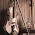How is A* algorithm different from AO* ? Out of the two which one is better and why?

1.A* Alg: Selects Best node on OPEN list with less Heuristic function. It contains only OR arcs., ie no need of dependencies in ancestors.
It contains both features of DFS and BFS.

AO*: Selects the Best node for generating new succors with less Heuristic value also part of Tp, if it is in AND arc.
It follows both AND and OR Arcs., Dependencies are considered in AND arcs.
IT follows Reason Backward search by adding Unit costs to calculate the Tp values of the node if it represents AND arc.

9.sir thank u so much

10.sir...its vry difficult to study AI, so pls suggst some tips to study and giv one gud exmple for AO* algorthm

1.A* Algorithm follows OR arcs., Bur AO* Algorithm follows both OR arcs and AND arcs., to select best node for generating new succor nodes in AO* Algorithm not only consider whether that is having less Heuristic Function value also calculate path value if that node is located in AND arc by propagating backword and add 1 unir cost to current f value i.w is shown above diagrams.,
then U can follow the above AO* search procedure. nothing difficult.

11.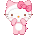how to solve 8- puzzle problem using BFS, DFS, HILL CLIMBING, BEST FIRST SEARCH.

please ive me the answer for that...i as waiting....

12.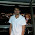very good article but here i am having a confusion in AO* algorithm's 2nd step you said
"(b) if successor is not a terminal node, mark it solved and assign zero to its h ' value.
(c) If successor is not a terminal node, compute it h' value."
isn't it wrong? how can you propose two different solutions for a same non terminal node?
Any Guidance you can give me will be greatly appreciated.
Thank You,

13.14.What is tp?

15.can i get a real time example which can be solved using ao* algorithm ?

16.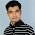Thank you 😊 so much sir..... Plz share all AI articles with me..

17.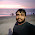thabnk you sir

18.Impressive!Thanks for the post
Artificial intelligence Solutions

19.Thank You For Sharing this information.
Artificial intelligence Solutions

20.This answer very helpful to me sir